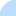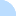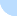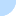## DebcheckDebcheck - bullseye  Summary  All packages (light) main only  Broken PreDepends  Broken Depends  Broken Recommends  Broken Suggests  HalfBroken PreDepends  HalfBroken Depends  HalfBroken Recommends  HalfBroken Suggests  Build-Depends  HalfBroken Build-Depends  Malformed Build-Depends  OldLibs  Priority  Old Standards-Version  No Standards-Version  Wrong Standards-Version Syntax other  Depends Main on !Main all sections  Broken PreDepends  Broken Depends  Broken Recommends  Broken Suggests  HalfBroken PreDepends  HalfBroken Depends  HalfBroken Recommends  HalfBroken Suggests  Build-Depends  HalfBroken Build-Depends  Malformed Build-Depends  OldLibs  Priority  Old Standards-Version  No Standards-Version  Wrong Standards-Version SyntaxDistribution: sid bullseye buster stretch jessie

# Problems for source package xsp

Current Source Version: 4.2-2.2
Current Maintainer: Debian Mono Group <pkg-mono-group@lists.alioth.debian.org>
Section: main
BTS entry: https://bugs.debian.org/src:xsp

# Binary Package: mono-xsp4 (Version: 4.2-2.2)

BTS entry: https://bugs.debian.org/mono-xsp4

### Relationships

Package has a Depends on mono-runtime (>= 3.0~) which cannot be satisfied on mips64el.

### Half Broken Relationships

(not necessarily bugs)

Package has a Depends on libc0.1 (>= 2.31) which cannot be satisfied on armel. At least one of libc6 (>= 2.31) | libc6.1 (>= 2.31) | libc0.1 (>= 2.31) can be satisfied however.
Package has a Depends on libc0.1 (>= 2.31) which cannot be satisfied on ppc64el. At least one of libc6 (>= 2.31) | libc6.1 (>= 2.31) | libc0.1 (>= 2.31) can be satisfied however.
Package has a Depends on libc0.1 (>= 2.31) which cannot be satisfied on mips64el. At least one of libc6 (>= 2.31) | libc6.1 (>= 2.31) | libc0.1 (>= 2.31) can be satisfied however.
Package has a Depends on libc0.1 (>= 2.31) which cannot be satisfied on amd64. At least one of libc6 (>= 2.31) | libc6.1 (>= 2.31) | libc0.1 (>= 2.31) can be satisfied however.
Package has a Depends on libc0.1 (>= 2.31) which cannot be satisfied on armhf. At least one of libc6 (>= 2.31) | libc6.1 (>= 2.31) | libc0.1 (>= 2.31) can be satisfied however.
Package has a Depends on libc0.1 (>= 2.31) which cannot be satisfied on mipsel. At least one of libc6 (>= 2.31) | libc6.1 (>= 2.31) | libc0.1 (>= 2.31) can be satisfied however.
Package has a Depends on libc0.1 (>= 2.31) which cannot be satisfied on arm64. At least one of libc6 (>= 2.31) | libc6.1 (>= 2.31) | libc0.1 (>= 2.31) can be satisfied however.
Package has a Depends on libc0.1 (>= 2.31) which cannot be satisfied on s390x. At least one of libc6 (>= 2.31) | libc6.1 (>= 2.31) | libc0.1 (>= 2.31) can be satisfied however.
Package has a Depends on libc0.1 (>= 2.31) which cannot be satisfied on i386. At least one of libc6 (>= 2.31) | libc6.1 (>= 2.31) | libc0.1 (>= 2.31) can be satisfied however.
Package has a Depends on libc6.1 (>= 2.31) which cannot be satisfied on i386. At least one of libc6 (>= 2.31) | libc6.1 (>= 2.31) | libc0.1 (>= 2.31) can be satisfied however.
Package has a Depends on libc6.1 (>= 2.31) which cannot be satisfied on s390x. At least one of libc6 (>= 2.31) | libc6.1 (>= 2.31) | libc0.1 (>= 2.31) can be satisfied however.
Package has a Depends on libc6.1 (>= 2.31) which cannot be satisfied on amd64. At least one of libc6 (>= 2.31) | libc6.1 (>= 2.31) | libc0.1 (>= 2.31) can be satisfied however.
Package has a Depends on libc6.1 (>= 2.31) which cannot be satisfied on mipsel. At least one of libc6 (>= 2.31) | libc6.1 (>= 2.31) | libc0.1 (>= 2.31) can be satisfied however.
Package has a Depends on libc6.1 (>= 2.31) which cannot be satisfied on armhf. At least one of libc6 (>= 2.31) | libc6.1 (>= 2.31) | libc0.1 (>= 2.31) can be satisfied however.
Package has a Depends on libc6.1 (>= 2.31) which cannot be satisfied on arm64. At least one of libc6 (>= 2.31) | libc6.1 (>= 2.31) | libc0.1 (>= 2.31) can be satisfied however.
Package has a Depends on libc6.1 (>= 2.31) which cannot be satisfied on armel. At least one of libc6 (>= 2.31) | libc6.1 (>= 2.31) | libc0.1 (>= 2.31) can be satisfied however.
Package has a Depends on libc6.1 (>= 2.31) which cannot be satisfied on ppc64el. At least one of libc6 (>= 2.31) | libc6.1 (>= 2.31) | libc0.1 (>= 2.31) can be satisfied however.
Package has a Depends on libc6.1 (>= 2.31) which cannot be satisfied on mips64el. At least one of libc6 (>= 2.31) | libc6.1 (>= 2.31) | libc0.1 (>= 2.31) can be satisfied however.

# Binary Package: mono-fpm-server (Version: 4.2-2.2)

BTS entry: https://bugs.debian.org/mono-fpm-server

### Relationships

Package has a Depends on libfpm-helper0 (>= 4.2-2.2) which cannot be satisfied on mips64el.
Package has a Depends on mono-runtime (>= 3.0~) which cannot be satisfied on mips64el.

# Binary Package: asp.net-examples (Version: 4.2-2.2)

BTS entry: https://bugs.debian.org/asp.net-examples

### Relationships

Package has a Depends on mono-runtime (>= 3.0~) which cannot be satisfied on mips64el.

# Binary Package: mono-apache-server4 (Version: 4.2-2.2)

BTS entry: https://bugs.debian.org/mono-apache-server4

### Relationships

Package has a Depends on mono-runtime (>= 3.0~) which cannot be satisfied on mips64el.

### Half Broken Relationships

(not necessarily bugs)

Package has a Depends on libc0.1 (>= 2.31) which cannot be satisfied on s390x. At least one of libc6 (>= 2.31) | libc6.1 (>= 2.31) | libc0.1 (>= 2.31) can be satisfied however.
Package has a Depends on libc0.1 (>= 2.31) which cannot be satisfied on i386. At least one of libc6 (>= 2.31) | libc6.1 (>= 2.31) | libc0.1 (>= 2.31) can be satisfied however.
Package has a Depends on libc0.1 (>= 2.31) which cannot be satisfied on mips64el. At least one of libc6 (>= 2.31) | libc6.1 (>= 2.31) | libc0.1 (>= 2.31) can be satisfied however.
Package has a Depends on libc0.1 (>= 2.31) which cannot be satisfied on ppc64el. At least one of libc6 (>= 2.31) | libc6.1 (>= 2.31) | libc0.1 (>= 2.31) can be satisfied however.
Package has a Depends on libc0.1 (>= 2.31) which cannot be satisfied on armel. At least one of libc6 (>= 2.31) | libc6.1 (>= 2.31) | libc0.1 (>= 2.31) can be satisfied however.
Package has a Depends on libc0.1 (>= 2.31) which cannot be satisfied on arm64. At least one of libc6 (>= 2.31) | libc6.1 (>= 2.31) | libc0.1 (>= 2.31) can be satisfied however.
Package has a Depends on libc0.1 (>= 2.31) which cannot be satisfied on mipsel. At least one of libc6 (>= 2.31) | libc6.1 (>= 2.31) | libc0.1 (>= 2.31) can be satisfied however.
Package has a Depends on libc0.1 (>= 2.31) which cannot be satisfied on armhf. At least one of libc6 (>= 2.31) | libc6.1 (>= 2.31) | libc0.1 (>= 2.31) can be satisfied however.
Package has a Depends on libc0.1 (>= 2.31) which cannot be satisfied on amd64. At least one of libc6 (>= 2.31) | libc6.1 (>= 2.31) | libc0.1 (>= 2.31) can be satisfied however.
Package has a Depends on libc6.1 (>= 2.31) which cannot be satisfied on s390x. At least one of libc6 (>= 2.31) | libc6.1 (>= 2.31) | libc0.1 (>= 2.31) can be satisfied however.
Package has a Depends on libc6.1 (>= 2.31) which cannot be satisfied on i386. At least one of libc6 (>= 2.31) | libc6.1 (>= 2.31) | libc0.1 (>= 2.31) can be satisfied however.
Package has a Depends on libc6.1 (>= 2.31) which cannot be satisfied on mips64el. At least one of libc6 (>= 2.31) | libc6.1 (>= 2.31) | libc0.1 (>= 2.31) can be satisfied however.
Package has a Depends on libc6.1 (>= 2.31) which cannot be satisfied on armel. At least one of libc6 (>= 2.31) | libc6.1 (>= 2.31) | libc0.1 (>= 2.31) can be satisfied however.
Package has a Depends on libc6.1 (>= 2.31) which cannot be satisfied on ppc64el. At least one of libc6 (>= 2.31) | libc6.1 (>= 2.31) | libc0.1 (>= 2.31) can be satisfied however.
Package has a Depends on libc6.1 (>= 2.31) which cannot be satisfied on arm64. At least one of libc6 (>= 2.31) | libc6.1 (>= 2.31) | libc0.1 (>= 2.31) can be satisfied however.
Package has a Depends on libc6.1 (>= 2.31) which cannot be satisfied on amd64. At least one of libc6 (>= 2.31) | libc6.1 (>= 2.31) | libc0.1 (>= 2.31) can be satisfied however.
Package has a Depends on libc6.1 (>= 2.31) which cannot be satisfied on mipsel. At least one of libc6 (>= 2.31) | libc6.1 (>= 2.31) | libc0.1 (>= 2.31) can be satisfied however.
Package has a Depends on libc6.1 (>= 2.31) which cannot be satisfied on armhf. At least one of libc6 (>= 2.31) | libc6.1 (>= 2.31) | libc0.1 (>= 2.31) can be satisfied however.

# Binary Package: mono-fastcgi-server4 (Version: 4.2-2.2)

BTS entry: https://bugs.debian.org/mono-fastcgi-server4

### Relationships

Package has a Depends on libfpm-helper0 (>= 4.2-2.2) which cannot be satisfied on mips64el.
Package has a Depends on mono-runtime (>= 3.0~) which cannot be satisfied on mips64el.

### Half Broken Relationships

(not necessarily bugs)

Package has a Depends on libc0.1 (>= 2.31) which cannot be satisfied on amd64. At least one of libc6 (>= 2.31) | libc6.1 (>= 2.31) | libc0.1 (>= 2.31) can be satisfied however.
Package has a Depends on libc0.1 (>= 2.31) which cannot be satisfied on armhf. At least one of libc6 (>= 2.31) | libc6.1 (>= 2.31) | libc0.1 (>= 2.31) can be satisfied however.
Package has a Depends on libc0.1 (>= 2.31) which cannot be satisfied on mipsel. At least one of libc6 (>= 2.31) | libc6.1 (>= 2.31) | libc0.1 (>= 2.31) can be satisfied however.
Package has a Depends on libc0.1 (>= 2.31) which cannot be satisfied on arm64. At least one of libc6 (>= 2.31) | libc6.1 (>= 2.31) | libc0.1 (>= 2.31) can be satisfied however.
Package has a Depends on libc0.1 (>= 2.31) which cannot be satisfied on armel. At least one of libc6 (>= 2.31) | libc6.1 (>= 2.31) | libc0.1 (>= 2.31) can be satisfied however.
Package has a Depends on libc0.1 (>= 2.31) which cannot be satisfied on ppc64el. At least one of libc6 (>= 2.31) | libc6.1 (>= 2.31) | libc0.1 (>= 2.31) can be satisfied however.
Package has a Depends on libc0.1 (>= 2.31) which cannot be satisfied on mips64el. At least one of libc6 (>= 2.31) | libc6.1 (>= 2.31) | libc0.1 (>= 2.31) can be satisfied however.
Package has a Depends on libc0.1 (>= 2.31) which cannot be satisfied on i386. At least one of libc6 (>= 2.31) | libc6.1 (>= 2.31) | libc0.1 (>= 2.31) can be satisfied however.
Package has a Depends on libc0.1 (>= 2.31) which cannot be satisfied on s390x. At least one of libc6 (>= 2.31) | libc6.1 (>= 2.31) | libc0.1 (>= 2.31) can be satisfied however.
Package has a Depends on libc6.1 (>= 2.31) which cannot be satisfied on i386. At least one of libc6 (>= 2.31) | libc6.1 (>= 2.31) | libc0.1 (>= 2.31) can be satisfied however.
Package has a Depends on libc6.1 (>= 2.31) which cannot be satisfied on s390x. At least one of libc6 (>= 2.31) | libc6.1 (>= 2.31) | libc0.1 (>= 2.31) can be satisfied however.
Package has a Depends on libc6.1 (>= 2.31) which cannot be satisfied on amd64. At least one of libc6 (>= 2.31) | libc6.1 (>= 2.31) | libc0.1 (>= 2.31) can be satisfied however.
Package has a Depends on libc6.1 (>= 2.31) which cannot be satisfied on mipsel. At least one of libc6 (>= 2.31) | libc6.1 (>= 2.31) | libc0.1 (>= 2.31) can be satisfied however.
Package has a Depends on libc6.1 (>= 2.31) which cannot be satisfied on armhf. At least one of libc6 (>= 2.31) | libc6.1 (>= 2.31) | libc0.1 (>= 2.31) can be satisfied however.
Package has a Depends on libc6.1 (>= 2.31) which cannot be satisfied on arm64. At least one of libc6 (>= 2.31) | libc6.1 (>= 2.31) | libc0.1 (>= 2.31) can be satisfied however.
Package has a Depends on libc6.1 (>= 2.31) which cannot be satisfied on armel. At least one of libc6 (>= 2.31) | libc6.1 (>= 2.31) | libc0.1 (>= 2.31) can be satisfied however.
Package has a Depends on libc6.1 (>= 2.31) which cannot be satisfied on ppc64el. At least one of libc6 (>= 2.31) | libc6.1 (>= 2.31) | libc0.1 (>= 2.31) can be satisfied however.
Package has a Depends on libc6.1 (>= 2.31) which cannot be satisfied on mips64el. At least one of libc6 (>= 2.31) | libc6.1 (>= 2.31) | libc0.1 (>= 2.31) can be satisfied however.

Last updated: Sat Jul 24 15:26:30 2021

DO NOT MASS FILE BUG REPORTS
GET A CONSENSUS ON debian-devel@lists.debian.org BEFORE MASS FILING BUGS